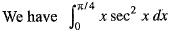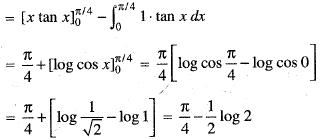Courses

# Integral Calculus -2

## 20 Questions MCQ Test Topic-wise Tests & Solved Examples for IIT JAM Mathematics | Integral Calculus -2

Description
This mock test of Integral Calculus -2 for Mathematics helps you for every Mathematics entrance exam. This contains 20 Multiple Choice Questions for Mathematics Integral Calculus -2 (mcq) to study with solutions a complete question bank. The solved questions answers in this Integral Calculus -2 quiz give you a good mix of easy questions and tough questions. Mathematics students definitely take this Integral Calculus -2 exercise for a better result in the exam. You can find other Integral Calculus -2 extra questions, long questions & short questions for Mathematics on EduRev as well by searching above.
QUESTION: 1

###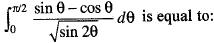Solution: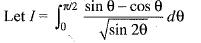...(i)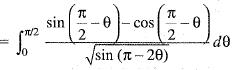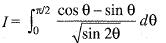....(ii)
Adding (i) and (ii), we get
2l=0
implies 1 = 0

QUESTION: 2

### The value of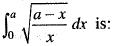Solution: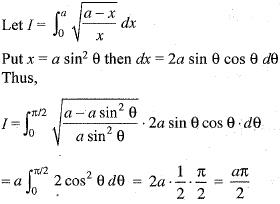QUESTION: 3

### The value of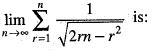Solution: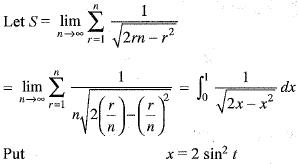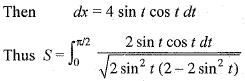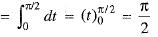QUESTION: 4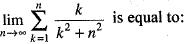Solution: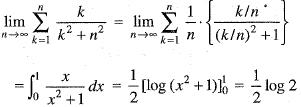QUESTION: 5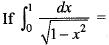mπ, then the value of m is:

Solution:

Given that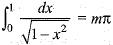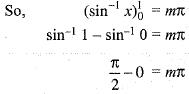Hence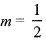QUESTION: 6

The value of the integral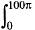| sin x | dx is equal to:

Solution: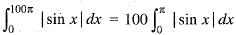[Since sin x is periodic with period π]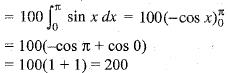QUESTION: 7

The value of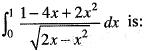Solution: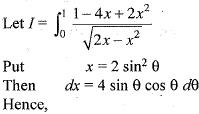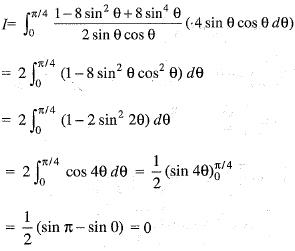QUESTION: 8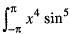x dx is equal to:

Solution: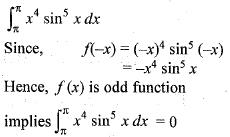QUESTION: 9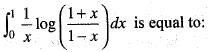Solution: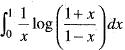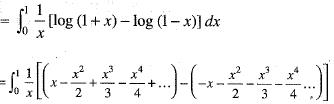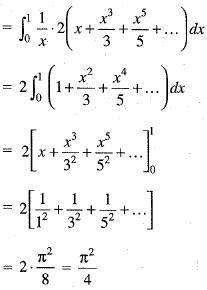QUESTION: 10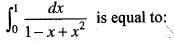Solution: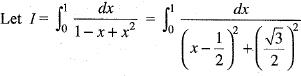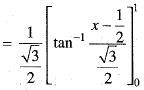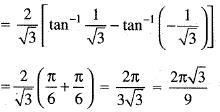QUESTION: 11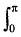xF(sin X) dx is equal to

Solution: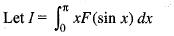...(i)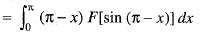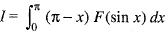...(ii)
Adding (i) and (ii), we get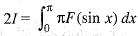Hence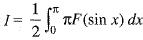QUESTION: 12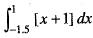= where [ ] represents greatest integer:

Solution: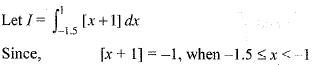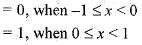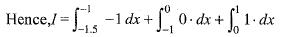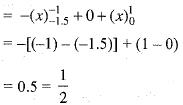QUESTION: 13

The value of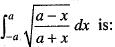Solution: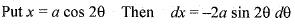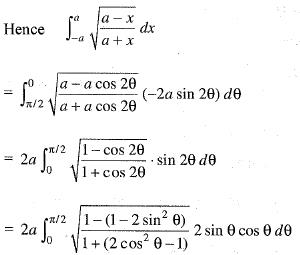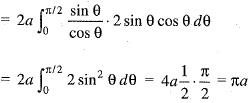QUESTION: 14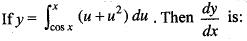Solution: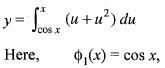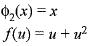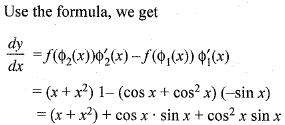QUESTION: 15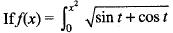dt, then the derivative of f(x) with respect to x is:

Solution: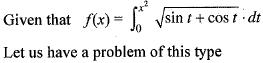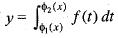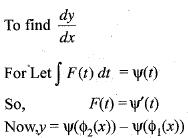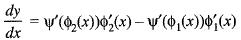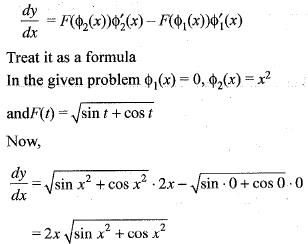QUESTION: 16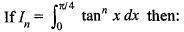Solution:

Given that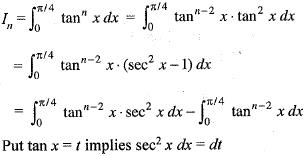Hence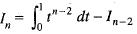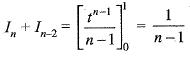QUESTION: 17

The value of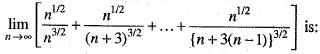Solution: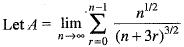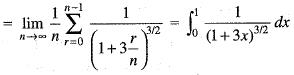QUESTION: 18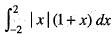is equal to

Solution: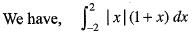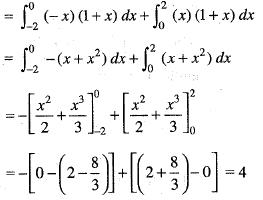QUESTION: 19

If f(x) is the integral of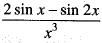, x ≠ 0, then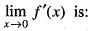Solution:

We have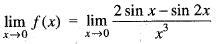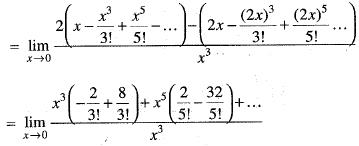QUESTION: 20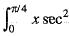x dx is equal to:

Solution: